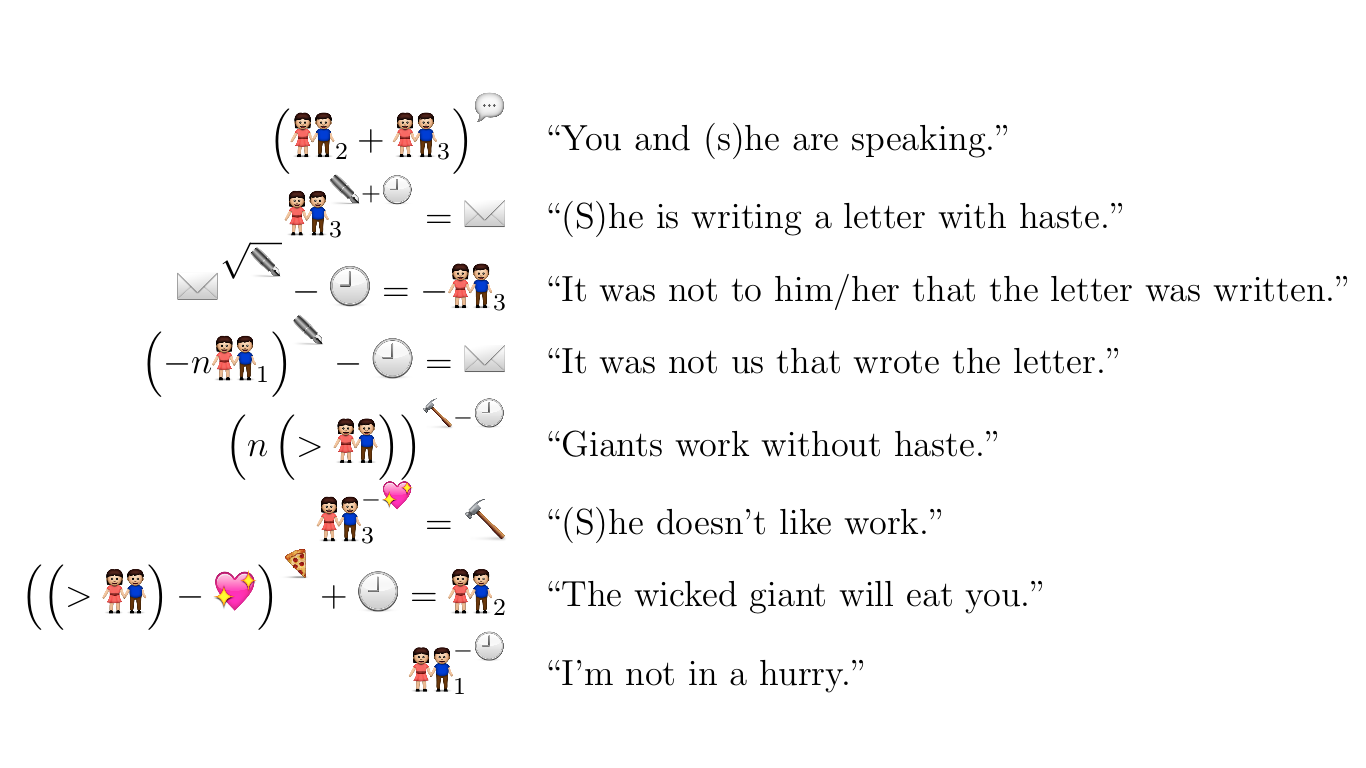The puzzlehunt has ended, thanks for playing!

# Emojebra```(person_2+person_3)^speech
person_3^(pen+clock)=letter
letter^sqrt(pen)-clock=-person_3
(-n*person_1)^pen-clock=letter
(n*(>person))^(hammer-clock)
person_3^(-heart)=hammer
((>person)-heart)^pizza+clock=person_2
person_1^(-clock)
```

Translate the following into emojebra:

• The amicable giant got eaten.
• The hungry midgets work unenthusiastically.
• The giant letter hastily ate the slow person.
• You are not loved by the talkative person.

Note: No spaces, standard precedence rules apply. Do not use unnecessary brackets.

## Solution

author: Alec Wright and Swaraj Dash

This is a linguistic puzzle where the candidate needs to understand the "grammar" system of Emojebra so that they can translate between English and Emojebra. From the example translations provided, the following patterns can be deciphered:

• "n" is used for plurals
• Subscripts indicate whether or not a pronoun is in the first, second, or third person
• "-" is used for negation (or any negative quality)
• The square root symbol is used for passive voice
• As an exponent, a symbol is a verb unless preceeded by a + or -, in which case it is an adverb
• A clock symbol as a base indicates the tense

Combining all these patterns, we can translate the given sentences to Emojebra:

• '(>person+heart)^sqrt(pizza)-clock' OR '((>person)+heart)^sqrt(pizza)-clock'
• '(n*(<person)-pizza)^(hammer-heart)'
• '(>letter)^(pizza+clock)-clock=person_3-clock' OR '(>letter)^(pizza+clock)-clock=person-clock'
• 'person_2^(-sqrt(heart))=person_3+speech' OR 'person_2^(-sqrt(heart))=person+speech'